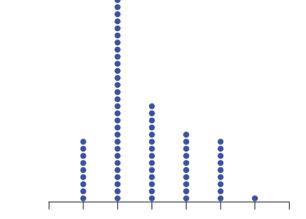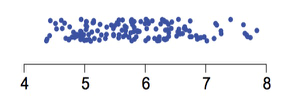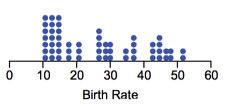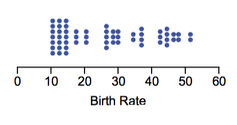# 语法

``chart.interval().position('x*y').color('x');``

type 说明
point 点，用于点图的构建。
path 路径，无序的点连接而成的一条线。
line 线，点按照 x 轴连接成一条线，构成线图。
area 填充线图跟坐标系之间构成区域图，也可以指定上下范围。
interval 使用矩形或者弧形，用面积来表示大小关系的图形，一般构成柱状图、饼图等图表。
polygon 多边形，可以用于构建热力图、地图等图表类型。
schema 自定义图形，用于构建箱须图、蜡烛图等图表。
heatmap 用于绘制热力图。
contour 用于绘制等高线。
edge 两个点之间的链接，用于构建树图中的边、流程图中的连接线。

type 说明
pointStack 层叠的点图
pointJitter 扰动点图
pointDodge 分组的点
intervalStack 层叠柱状图
intervalDodge 分组柱状图
intervalSymmetric 对称的柱状图，多用于绘制漏斗图。
areaStack 层叠面积图

• stack 层叠• jitter 将点抖开• dodge 分组• symmetric 对称## 属性

【可选】，声明几何对象图形的数据调整方式，主要用于绘制层叠图、扰动图、分组图等。可支持的数据格式为 String 字符串或者 Array 数据（表示多种调整方式组合）。

pointStack chart.point('stack')
pointJitter chart.point('jitter')
pointDodge chart.point(['dodge', 'stack'])
intervalStack chart.interval('stack')
intervalDodge chart.interval('dodge')
intervalSymmetric chart.interval('symmetric')
areaStack chart.area('stack')

## 方法

### position

`position(dims)`

#### chart.geom().position(dim)

dim 为字符串，代表数据源中某一个字段，用于一维坐标系图表绘制。 示例

#### chart.geom().position(Stat.summary.median('dimA*dimB'))

##### 示例
``````chart.point().position('cut');
chart.interval().position('cut*price');
chart.interval().position(Stat.summary.median('cut*price'));``````

### color

`color(dim, colors)`

#### chart.geom().color(dim | color);

##### 参数
• `dim` or `color`

只支持接收一个参数，dim 可以为映射至颜色属性的数据源字段名，如果数据源中不存在这个字段名的话，则按照常量进行解析，也可以直接指定某一个具体的颜色值 color，如 '#fff', 'white' 等。

##### 示例
``````chart.point().position('x*y').color('x');
chart.point().position('x*y').color('red')``````

#### chart.geom().color(dim[, colors]);

##### 参数
• `dim`

dim 为映射至颜色属性的数据源字段名

• `colors`

colors 的参数有以下情况：

• 如果为空，即未指定颜色的数组，那么使用内置的全局的颜色；
• 如果需要指定颜色，则需要以数组格式传入，那么分类的颜色按照数组中的颜色确定。对于颜色的分配顺序，会默认按照原始数据源中字段的顺序进行分配；
• 还支持指定颜色的计算方式，目前 G2 默认提供了多种的计算方式：'red', 'blue', 'green', 'rainbow', 'hue', 'lightness', 'brightness'，常用于映射连续类型数据的颜色；
• 还支持这种格式：`color1-color2`，用于指定一个渐变色，数据根据分类或者连续类型，在渐变的颜色区间内取颜色。
##### 示例
``````chart.point().position('x*y').color('z'); // 使用默认的颜色
chart.point().position('x*y').color('z', ['red', 'blue']); // 使用传入的指定颜色
chart.point().position('x*y').color('z', 'hue'); // 使用某种颜色计算方式，这里是使用色调
chart.point().position('x*y').color('z','red-blue'); // 使用渐变色``````

#### chart.geom().color(dim, colorCallback);

##### 参数
• `dim`

dim 为映射至颜色属性的数据源字段名

• `colorCallback`

[Function]，指定一个颜色的回调函数，用于高灵活度的用户自定义。

##### 示例
``````chart.point().position('x*y').color('z', function(value) {
if(value === 1) {
return 'red'
}else {
return 'blue';
}
});``````

### shape

`shape(dim, shapes)`

#### chart.geom().shape('shape');

##### 参数
• `shape`

只支持接收一个参数，指定几何图像对象绘制的形状。下表列出了不同的 geom 几何图形对象支持的 shape 形状：

geom 类型 shape 类型 解释
point 'circle','square','bowtie','diamond','hexagon','triangle','triangle-down',
'hollowCircle','hollowSquare','hollowBowtie','hollowDiamond',
'hollowHexagon','hollowTriangle','hollowTriangle-down',
'cross','tick','plus','hyphen','line','rect'
hollow开头的图形都是空心的
line 'line','smooth','dot','dash','dotSmooth','spline' dot ：点线，smooth： 平滑线,spline:限定了范围的平滑线
area 'area','smooth','line','dotLine','smoothLine','dotSmoothLine' areasmooth是填充内容的区域图，其他图表是空心的线图
interval 'rect','hollowRect','line','tick','stroke' hollowRect:空心的矩形， line 和 tick 都是线段,stroke：带边框的矩形
polygon 'polygon','hollow','stroke' polygon：多边形、hollow：空心多边形和 stroke：带边框的多边形
schema 'box','candle' 目前仅支持箱须图、K线图
edge 'line','vhv','smooth','arc' vhv：直角折线，arc：弧线，分为笛卡尔坐标系、极坐标系、带权重和不带权重四种情况，具体使用如下表
##### 示例
``chart.point().position('x*y').shape('square'); // 指定所有点的图形是正方形``

#### chart.geom().shape(dim, shapes);

##### 参数
• `dim`

dim 为映射至颜色属性的数据源字段名。

• `shapes`

shapes 是一个可选参数，如果没有声明会按照 G2 默认为特定 geom 类型配置的形状进行渲染，当然用户也可自己指定渲染的形状，具体的形状已在上面列出，下面是 G2 为特定的几何图形对象提供的 shapes:

``````var shapes = {
point: ['hollowCircle', 'hollowSquare', 'hollowDiamond', 'hollowBowtie', 'hollowTriangle',
'hollowHexagon', 'cross', 'tick', 'plus', 'hyphen', 'line'
],
line: ['line', 'dash', 'dot'],
area: ['area']
};``````
##### 示例
``````var defs = {
'cut': {
values: ['Ideal', 'Premium', 'Very-Good', 'Good', 'Fair']
}
};
chart.source(data, defs);
chart.point().position('carat*price').shape('cut'); // 使用默认的 shapes
chart.point().position('carat*price').shape('cut', ['cross', 'tick', 'plus', 'hyphen', 'line']); // 使用自定义的 shapes``````

#### chart.geom().shape(dim, callback);

##### 参数
• `dim`

dim 为映射至颜色属性的数据源字段名。

• `callback`

[Function] 回调函数

##### 示例
``````chart.point().position('x*y').shape('z', function(value) {
if (value === 1) {
return 'circle'
}else {
return 'square';
}
});``````

### size

`size(dim, max, min)`

#### chart.geom().size(value）

• point 图形的 size 影响点的半径大小；
• line, area, path 中的 size 影响线的粗细；
• interval 的 size 影响柱状图的宽度。

#### chart.geom().size(dim)

##### 示例
``chart.point().position('x*y').size('z'); // 使用 z 字段的值来映射大小``

#### chart.geom().size(dim, max, min)

##### 示例
``````chart.point().position('x*y').size('z', 100); // 使用 z 字段的值来映射大小，最大值为 100，使用默认的最小值 1
chart.point().position('x*y').size('z', 100, 10); // 使用 z 字段的值来映射大小，最大值为 100，最小值 10``````

#### chart.geom().size(dim, callback)

##### 示例
``````chart.point().position('x*y').size('z', function(value) {
if(value === 1) {
return 5;
}
return 10;
});``````

### opacity

`opacity(dim[, callback])`

#### chart.geom().opacity(value)

##### 示例
``chart.interval().position('x*y').opacity(0.8); // 图形颜色为 0.8 透明度``

#### chart.geom().opacity(dim)

##### 示例
``chart.interval().position('x*y').opacity('z');``

#### chart.geom().opacity(dim, callback)

##### 示例
``````chart.point().position('x*y').opacity('z', function(value) {
if(value === 1) {
return 0.5;
}
return 0.8;
});``````

### label

`label(dim[, cfg])`

#### chart.geom().label(dim)

##### 示例
``chart.point().position('x*y').label('x');``

#### chart.geom().label(dim, cfg)

##### 参数
• `cfg`
``````chart.intervalStack().position('x*y').label('x', {
offset: -16, // 文本距离图形的距离
lableLine: false, //  是否显示点到图形的连接线，目前仅饼图支持，默认为 true，显示连接线
label: {
fontWeight: 'bold', // 文本粗细
fontFamily: '宋体', // 文本字体
fontSize: 12, // 文本大小
rotate: Math.PI, // 文本旋转的角度，一般自动计算
textAlign: 'center' // 文本对齐方式，可取值 left center right，默认为 center
}, // 所有文本共享的配置信息
custom: false, // 是否使用自定义文本，如果是true，那么就使用html显示文本
renderer: function(text, item, index)  {
// 配合 custom 为 true 使用，格式化文本的函数
}
});``````
##### 示例
``````// 格式化饼图文本
function formatter(text, item) {
var point = item.point; // 每个弧度对应的点
var proportion = point['..proportion']; // ..proportion 字段由Stat.summary.proportion统计函数生成
proportion = (proportion * 100).toFixed(2) + '%';
return '<span class="title">' + text + '</span><br><span style="color:' + point.color + '">' + proportion + '</span>';
}
\$.getJSON('../static/data/diamond.json',function (data) {
var Stat = G2.Stat;
var chart = new G2.Chart({
id: 'c1',
width: 800,
height: 400,
plotCfg: {
margin: [20, 90, 60, 80]
}
});
chart.source(data);
// 不同cut（切割工艺）所占的比例
chart.intervalStack()
.position(Stat.summary.proportion())
.color('cut')
.label('cut', {
custom: true, // 使用自定义文本
renderer: formatter, // 格式化文本的函数
labelLine: false, // 不显示文本的连接线
offset: 50 // 文本距离图形的距离
});
chart.render();
});``````

#### chart.geom().label(dim, callback, labelCfg)

##### 示例
``````chart.polygon().position(Stat.treemap('children*value')).color('type').shape('stroke')
.label('name*children', function(name, children) { // 仅显示没有子节点的名称
if (!children) {
return name;
}
}, {
label: {
fill: '#fff'
}
});``````

### tooltip

`tooltip(dim)`

##### 参数
• `dim`

对应数据源的某个字段，可以指定一个或者多个，当有多个时，使用 * 来连接。

##### 示例
``````var data = [
{"gender":"female","height":161.2,"weight":51.6},
{"gender":"female","height":167.5,"weight":59},
{"gender":"male","height":159.5,"weight":49.2},
{"gender":"male","height":157,"weight":63},
{"gender":"female","height":155.8,"weight":53.6}
];
var chart = new G2.Chart({
id: 'c2',
width : 800,
height : 400
});

chart.source(data, {
weight: {
alias: '体重（kg）'
},
height: {
alias: '身高（cm）'
}
});
chart.tooltip({
title: null, // 在这里设置不显示 title
map: {
name: 'height',
value: 'weight'
} // 这个 map 属性被覆盖了
});
chart.point().position('height*weight')
.color('gender', ['#f96a52', '#00a3d7'])
.opacity(0.5)
.shape('circle')
.tooltip('gender*height*weight');
chart.render();``````

### style

`style(cfg)`

##### 参数
``````var cfg = {
point: {
lineWidth: 1,
fill: '#999',
},
hollowPoint: {
fill: '#fff',
lineWidth: 1,
stroke: '#999',
},
interval: {
lineWidth: 0,
fill: '#999',
fillOpacity: 0.85
},
strokeInterval: {
lineWidth: 1,
stroke: '#fff'
},
pie: {
lineWidth: 1,
stroke: '#fff'
},
hollowInterval: {
fill: '#fff',
stroke: '#999',
fillOpacity: 0,
lineWidth: 1
},
area: {
lineWidth: 0,
fill: '#999',
fillOpacity: 0.6
},
polygon: {
lineWidth: 0,
fill: '#999',
fillOpacity: 1
},
hollowPolygon: {
fill: '#fff',
stroke: '#999',
fillOpacity: 0,
lineWidth: 1
},
strokePolygon: {
fill: '#999',
stroke: '#efefef',
fillOpacity: 1
},
hollowArea: {
fill: '#fff',
stroke: '#999',
fillOpacity: 0,
lineWidth: 1
},
line: {
stroke: '#999',
lineWidth: 1,
fill: null
}
};``````

### selected

`selected(enable, cfg)` 用于设置几何标记的选中模式。

``````// 关闭选中模式
chart.<geom>().position('x*y').selected(false); // 方式 1
chart.<geom>().position('x*y').selected({
selectedMode: false
}); // 方式 2

// 开启选中模式, true 可省略
chart.<geom>().position('x*y').selected(true, {
selectedMode: 'multiple' || 'single', // multiple 为多选，single 为单选
style: {
// 设置选中图形的样式，不设置则使用默认提供的样式
}
});``````

#### 参数

• `enable`: Boolean

• `cfg`: Object

``````{
selectedMode: false | 'multiple' | 'single', // 是否可选中、多选、单选
style: {
// 绘图属性
}, // 设置选中图形的样式，不设置则使用默认提供的样式
cancelable: true | false, // 选中之后是否可取消选中
animate: true | false // 选中是否执行动画
}``````